# GMAT Math : DSQ: Calculating the perimeter of an acute / obtuse triangle

## Example Questions

2 Next →

### Example Question #16 : Acute / Obtuse Triangles

What is the perimeter of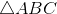?

Statement 1: The triangle with its vertices at the midpoints of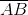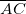, and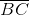has perimeter 34.

Statement 2:, andare the midpoints of the sides of a triangle with perimeter 136.

Statement 2 ALONE is sufficient to answer the question, but Statement 1 ALONE is NOT sufficient to answer the question.

EITHER statement ALONE is sufficient to answer the question.

BOTH statements TOGETHER are insufficient to answer the question.

Statement 1 ALONE is sufficient to answer the question, but Statement 2 ALONE is NOT sufficient to answer the question.

BOTH statements TOGETHER are sufficient to answer the question, but NEITHER statement ALONE is sufficient to answer the question.

EITHER statement ALONE is sufficient to answer the question.

Explanation:

Assume Statement 1 alone. A segment that has as its endpoints the midpoints of two sides of a triangle is a midsegment of the triangle, and its length is half that of the side to which it is parallel. Therefore, the sum of the lengths of the midsegments—that is, the perimeter of the triangle they form, which from Statement 1 is 34, is half the perimeter of the larger triangle, which here is. The perimeter ofis therefore 68.

Assume Statement 2 alone. Here,itself is the triangle formed by the midsegments. Since the larger triangle has perimeter 136,has perimeter half this, or 68.

### Example Question #17 : Acute / Obtuse Triangles

True or false: The perimeter ofis greater than 60.

Statement 1:is an isosceles triangle.

Statement 2: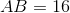and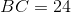EITHER statement ALONE is sufficient to answer the question.

Statement 1 ALONE is sufficient to answer the question, but Statement 2 ALONE is NOT sufficient to answer the question.

BOTH statements TOGETHER are sufficient to answer the question, but NEITHER statement ALONE is sufficient to answer the question.

BOTH statements TOGETHER are insufficient to answer the question.

Statement 2 ALONE is sufficient to answer the question, but Statement 1 ALONE is NOT sufficient to answer the question.

BOTH statements TOGETHER are insufficient to answer the question.

Explanation:

Assume both statements to be true. An isosceles triangle has two sides of equal length, so either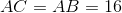or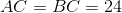. By the Triangle Inequality Theorem, the length of each side must be less than the sum of the lengths of the other two; both scenarios are possible, since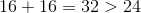and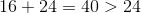.

If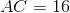, the perimeter ofis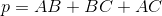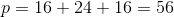.

If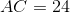, the perimeter ofis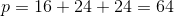.

Without further information, it is impossible to determine whether the perimeter is less than or greater than 60.

### Example Question #18 : Acute / Obtuse Triangles

What is the perimeter of?

Statement 1: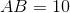and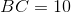Statement 2: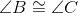BOTH statements TOGETHER are sufficient to answer the question, but NEITHER statement ALONE is sufficient to answer the question.

EITHER statement ALONE is sufficient to answer the question.

Statement 1 ALONE is sufficient to answer the question, but Statement 2 ALONE is NOT sufficient to answer the question.

BOTH statements TOGETHER are insufficient to answer the question.

Statement 2 ALONE is sufficient to answer the question, but Statement 1 ALONE is NOT sufficient to answer the question.

BOTH statements TOGETHER are sufficient to answer the question, but NEITHER statement ALONE is sufficient to answer the question.

Explanation:

From Statement 1 alone, we know that two sides are of length 10, but we are not given any clue as to the length of the third. Therefore, we cannot calculate the sum of the side lengths, which is the perimeter. Statement 2 alone is unhelpful, since it only gives that two angles have equal measure; applying the Converse of the Isosceles Triangle Theorem, it can be determined that their opposite sidesandare congruent, but no actual side lengths can be found.

Now assume both statements to be true. From Statement 1,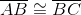, and, as stated before, it follows from Statement 2 that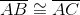. Therefore, the triangle is equilateral with sides of common length 10, making the perimeter 30.

2 Next →

Tired of practice problems?

Try live online GMAT prep today.